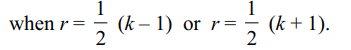## Permutations and Combinations Questions and Answers Part-6

1. Let an, bn, $n\epsilon N$   be defined as follows :
$a_{1}=1,a_{1}+2a_{2}+....+na_{n}=a_{n+1}\forall n\geq 1$
and $b_{1}=1,b_{1}+\frac{1}{2}b_{2}+....+\frac{1}{n}b_{n}=b_{n+1}\forall n\geq 2$         , then
a) $a_{n}=\frac{1}{2}\left(n!\right)\forall n\geq 2$
b) $b_{n}=\frac{1}{2}n\forall n\geq 2$
c) $\sum_{k=1}^{n}a_{k}b_{k}=\frac{1}{4}\left(\left(n+1\right)!+2\right)$
d) All of the above

Explanation: For n ≥ 1,2. A class has 30 students. The following prizes are to be awarded to the students of this class. First and second in Mathematics; first and second in Physics first in Chemistry and first in Biology. If N denote the number of ways in which this can be done, then
a) $400\mid N$
b) $600\mid N$
c) $8100\mid N$
d) All of the above

Explanation: First and second prizes in Mathematics (Physics) can be awarded in $^{30}P_{2}$ ( $^{30}P_{2}$ ) ways. First prize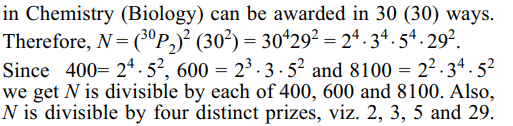3. A letter lock consists of three rings marked with 15 different letters. If N denotes the number of ways in which it is possible to make unsuccessful attempts to open the lock, then
a) $482\mid N$
b) N is product of 2 distinct prime numbers
c) N is product of 3 distinct prime numbers
d) Both a and c

Explanation: Since each ring has 15 positions, the total number of attempts that can be made to open the lock is 153 . Out of these, there is just one attempt in which the lock will open. Therefore,4. $^{2n}P_{n}$  is equal to
a) (n + 1) (n + 2).... (2n)
b) $2^{n}\left[1.3.5 .... \left(2n-1\right)\right]$
c) (2) . (6) . (10).... (4n – 2)
d) All of the above

Explanation: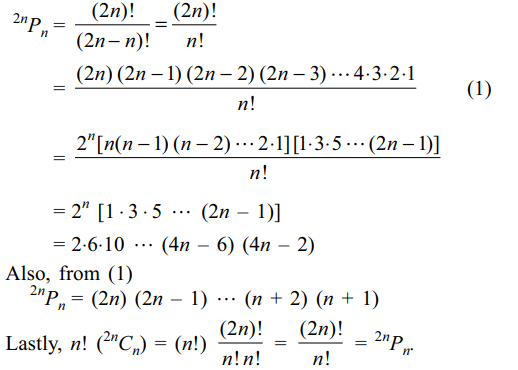5.If n objects are arranged in a row, then the number of ways of selecting three of these objects so that no two of them are next to each other is
a) $\frac{1}{6}\left(n-2\right)\left(n-3\right)\left(n-4\right)$
b) $^{n-2}C_{3}$
c) $^{n-3}C_{3}+^{n-3}C_{2}$
d) All of the above

Explanation: Let x0 be the number of objects to the left of the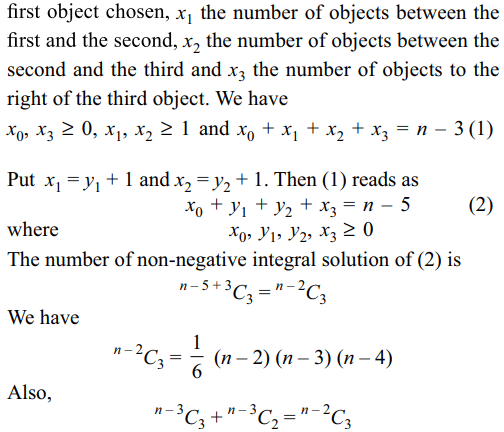6.The number of non-negative integral solutions of $x_{1}+x_{2}+x_{3}+x_{4}\leq n$     (where n is a positive integer) is
a) $^{n+3}C_{3}$
b) $^{n+4}C_{4}$
c) $^{n+4}C_{n}$
d) Both b and c

Explanation: Let x5 be such that x1 + x2 + x3 + x4 + x5 = n. We now seek the non-negative integral solutions of x1 + x2 + x3 + x4 + x5 = n. The number of required solutions = $^{n+4}C_{n}$ = $^{n+4}C_{4}$

7. The number of ways of choosing triplets (x, y, z) such that z ≥ max {x, y} and $x,y,z \epsilon \left\{1,2,....,n,n+1\right\}$     is
a) $^{n+1}C_{3}+^{n+2}C_{3}$
b) $\frac{1}{6}n\left(n+1\right) \left(2n +1\right)$
c) $1^{2}+2^{2}+....+n^{2}$
d) All of the above

Explanation: When z = n + 1, we can choose x, y from8. If $100!=2^{\alpha}3^{\beta}5^{\gamma}7^{\delta}....$     , then
a) $\alpha=97$
b) $\beta=\frac{1}{2}\left(\alpha-1\right)$
c) $\gamma=\frac{1}{2}\beta$
d) All of the above

Explanation: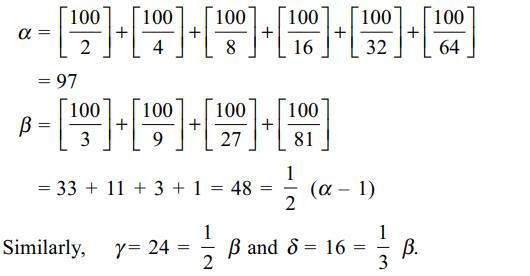9. The number of triangles that can be formed with the sides of lengths a, b and c where a, b, c are integers such that $a\leq b\leq c$   is
a) $\frac{1}{4}\left(c+1\right)^{2}$   when c is odd
b) $\frac{1}{2}c\left(c+1\right)$   when c is odd
c) $\frac{1}{4}c\left(c+2\right)$   when c is even
d) Both a and c10. If k is odd then $^{k}C_{r}$ is maximum for r equal to
a) $\frac{1}{2}\left(k-1\right)$
b) $\frac{1}{2}\left(k+1\right)$
Explanation: Let k = 2n + 1, then $^{2n+1}C_{r}$ is maximum when r = n. Also $^{2n+1}C_{n}$ = $^{2n+1}C_{n+1}$. Thus, $^{k}C_{r}$ is maximum